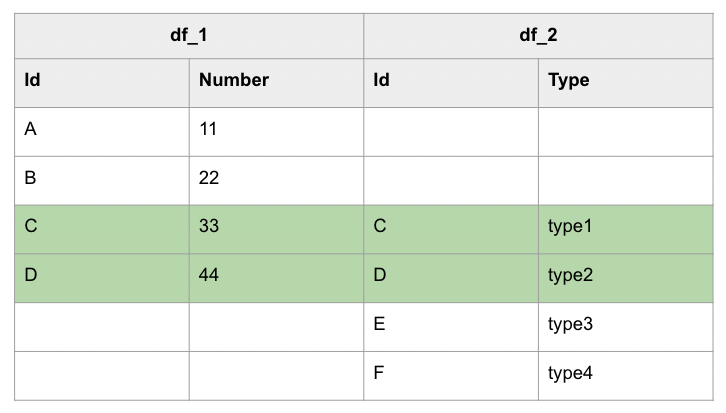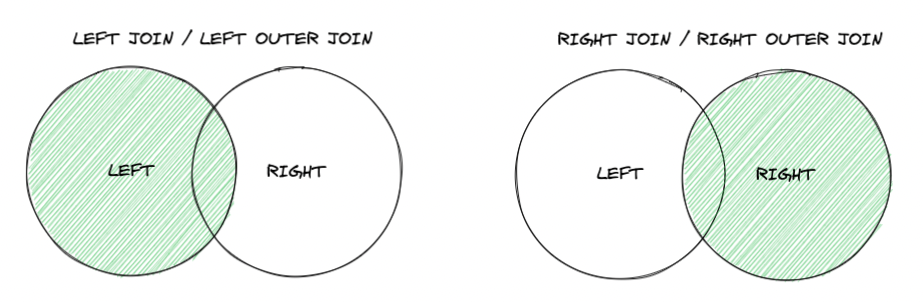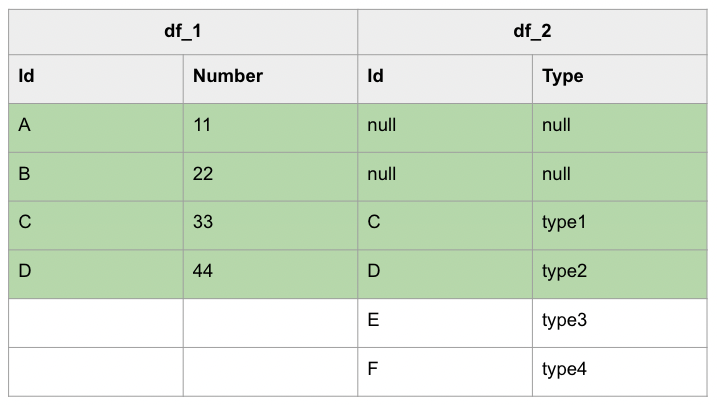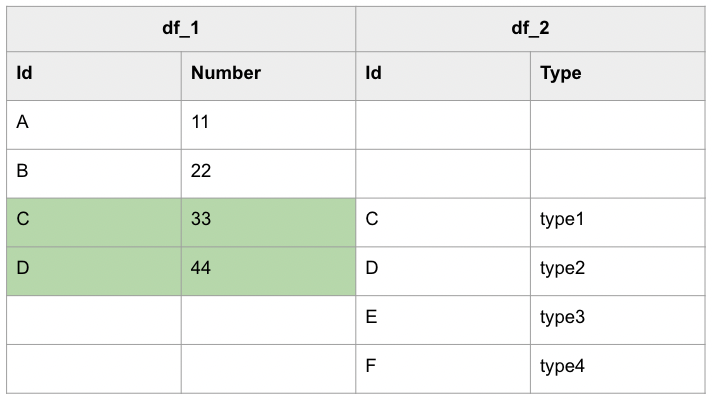# 零經驗也可的 PySpark 教學 - DataFrame part 2

Posted on  Dec 16, 2022  in  Python 模組/套件推薦 , Python 程式設計 - 高階  by  Amo Chen  ‐ 8 min read

### 測試資料集 #

``````\$ cd nyt-comments/
``````

### 啟動 PySpark Notebook Docker Container 指令 #

``````\$ docker run -it -p 8888:8888 -v \$PWD:/home/jovyan jupyter/pyspark-notebook
``````

### JOIN #

RDBMS(Relational Database Management System)提供 SQL JOIN 的功能，讓我們能夠將不同表格資料之間透過關聯(relation)結合起來，同樣地 Spark 也提供 JOIN 的方式將不同的資料結合起來。

1. INNER JOIN
2. LEFT JOIN / LEFT OUTER JOIN
3. RIGHT JOIN / RIGHT OUTER JOIN
4. LEFT ANTI JOIN
5. LFET SEMI JOIN
6. CROSS JOIN#### 將 Pandas DataFrame 轉換為 PySpark DataFrame #

``````import pandas as pd
from pyspark.sql import SparkSession

dataset_1 = [['A', 11], ['B', 22], ['C', 33],['D', 44]]
dataset_2 = [['C', 'type1'], ['D','type2'], ['E', 'type3'],['F','type4']]

pandas_df1 = pd.DataFrame(dataset_1, columns = ['Id', 'Number'])
pandas_df2 = pd.DataFrame(dataset_2, columns = ['Id', 'Type'])

spark = SparkSession.builder.getOrCreate()
df_1 = spark.createDataFrame(pandas_df1)
df_2 = spark.createDataFrame(pandas_df2)
``````#### INNER JOIN #

INNER JOIN 只會將有被關聯起來的資料羅列出來。

``````df_1.join(df_2, df_1.Id == df_2.Id, 'inner')\
.select(df_1.Id, df_1.Number, df_2.Type)\
.show()
``````

``````+---+------+-----+
| Id|Number| Type|
+---+------+-----+
|  C|    33|type1|
|  D|    44|type2|
+---+------+-----+
``````#### LEFT JOIN / RIGHT JOIN #

INNER JOIN 只會將有被關聯起來的資料羅列出來，但如果有些資料不能被關聯起來，但仍要羅列出來的話，就可以使用 LEFT JOIN 或者 RIGHT JOIN 進行操作，而無法被關聯起來的資料欄位就會以 `null` 代替，光看文字敘述的話仍無法深刻理解何為 LEFT JOIN 或 RIGHT JOIN, 畫成圖之後將會更好理解，如下圖所示：LEFT 與 RIGHT 的差異只在於要以哪一邊的資料為準，也就是上圖中的左邊圈圈或是右邊圈圈。

LEFT JOIN:RIGHT JOIN:``````df_1.join(df_2, df_1.Id == df_2.Id, 'left')\
.select(df_1.Id, df_1.Number, df_2.Type)\
.show()
``````

``````+---+------+-----+
| Id|Number| Type|
+---+------+-----+
|  A|    11| null|
|  B|    22| null|
|  C|    33|type1|
|  D|    44|type2|
+---+------+-----+
``````

``````df_1.join(df_2, df_1.Id == df_2.Id, 'right')\
.select(df_1.Id, df_1.Number, df_2.Type)\
.collect()
``````

``````df_2.join(df_1, df_2.Id == df_1.Id, 'left')\
.select(df_2.Id, df_1.Number, df_2.Type)\
.collect()
``````

``````+----+------+-----+
|  Id|Number| Type|
+----+------+-----+
|   C|    33|type1|
|   D|    44|type2|
|null|  null|type3|
|null|  null|type4|
+----+------+-----+
``````

#### OUTER JOIN #

OUTER JOIN 也被稱為 FULL JOIN 或 FULL OUTER JOIN, 其 JOIN 結果會無視是否關聯成功，將所有資料都羅列出來，就如同下圖所示：``````df_1.join(df_2, df_1.Id == df_2.Id, 'full')\
.select(df_1.Id, df_1.Number, df_2.Type)\
.collect()
``````

``````+----+------+-----+
|  Id|Number| Type|
+----+------+-----+
|   A|    11| null|
|   B|    22| null|
|   C|    33|type1|
|   D|    44|type2|
|null|  null|type3|
|null|  null|type4|
+----+------+-----+
``````

#### LEFT SEMI JOIN #

A semi join returns values from the left side of the relation that has a match with the right. It is also referred to as a left semi join. LEFT SEMI JOIN 與 INNER JOIN 相似，不過 SEMI JOIN 只會保留 LEFT 的資料，例如下圖：``````df_1.join(df_2, df_1.Id == df_2.Id, 'leftsemi')\
.show()
``````

``````+---+------+
| Id|Number|
+---+------+
|  C|    33|
|  D|    44|
+---+------+
``````

#### LEFT ANTI JOIN #

An anti join returns values from the left relation that has no match with the right. It is also referred to as a left anti join.

LEFT ANTI JOIN 會將無法關聯起來的資料羅列出來。``````df_1.join(df_2, df_1.Id == df_2.Id, 'leftanti')\
.show()
``````

``````+---+------+
| Id|Number|
+---+------+
|  A|    11|
|  B|    22|
+---+------+
``````

#### 用 SQL 語法進行 JOIN 操作 #

``````from pyspark.sql import SparkSession

spark = SparkSession.builder.getOrCreate()

df_1.createOrReplaceTempView('df_1')
df_2.createOrReplaceTempView('df_2')

joined_df = spark.sql('''
select * from df_1
inner join df_2
where df_1.Id == df_2.Id
''')

joined_df.show(truncate=False)
``````

``````+---+------+---+-----+
|Id |Number|Id |Type |
+---+------+---+-----+
|C  |33    |C  |type1|
|D  |44    |D  |type2|
+---+------+---+-----+
``````

#### 使用真實資料集進行 JOIN #

``````from pyspark.sql import SparkSession

spark = SparkSession.builder.getOrCreate()

.option('sep', ',')\
.option('escape', '"')\
.option('inferSchema', True)\
.option('multiline', True)\

.option('sep', ',')\
.option('escape', '"')\
.option('inferSchema', True)\
.option('multiline', True)\
.csv('/home/jovyan/ArticlesFeb2017.csv')
``````

INNER JOIN 範例：

``````df1.filter(df1.userID == '67892453')\
.join(df2, df1.articleID == df2.articleID, 'inner')\
collect()
``````

LEFT JOIN 範例：

``````df1.filter(df1.userID == '67892453')\
.join(df2, df1.articleID == df2.articleID, 'left')\
.collect()
``````

RIGHT JOIN 範例：

``````df1.filter(df1.userID == '67892453')\
.join(df2, df1.articleID == df2.articleID, 'right')\
.collect()
``````

### Alias 別名 #

PySpark 的欄位(column)提供 alias() 方法，讓我們能夠在查詢時，給欄位指定個別名(alias)以方便我們賦予欄位更清楚的名字或者方便我們後續用別名操作欄位，譬如下列範例將 `replyCount` 指定別名為 `reply_count`

``````df1.select(
).show(1)
``````

``````+-----------+
+-----------+
|          0|
+-----------+
``````

``````df1.createTempView('df1')

spark.sql('''
select
from
df1
''').show(1)
``````

``````df1.select(
).show(1)
``````

``````+-------------+
+-------------+
|         1000|
+-------------+
``````

``````spark.sql('''
select
from
df1
''').show(1)
``````

### GROUP BY #

GROUP BY 也是經常使用的 SQL 操作之一。

PySpark 的 DataFrame 也提供 groupBy() 方法，讓我們進行前述的操作，例如下列範例，我們想知道每個 New York Times comment 資料集的 commentType 與其相對應的資料筆數，可以使用 groupBy 搭配 count 進行：

``````from pyspark.sql import SparkSession

spark = SparkSession.builder.getOrCreate()

.option('sep', ',')\
.option('escape', '"')\
.option('inferSchema', True)\
.option('multiline', True)\

df.groupBy([df.commentType]).count().show()
``````

``````+-------------+------+
|  commentType| count|
+-------------+------+
|      comment|181750|
+-------------+------+
``````

``````from pyspark.sql.functions import desc

df.groupBy([
df.commentType
]).count().orderBy(desc('count')).show()
``````

p.s. orderBy 方法是 sort() 的別名(alias)，兩者是相同的方法

``````+-------------+------+
|  commentType| count|
+-------------+------+
|      comment|181750|
+-------------+------+
``````

``````from pyspark.sql.functions import asc

df.groupBy([
df.commentType
]).count().orderBy(asc('count')).show()
``````
``````+-------------+------+
|  commentType| count|
+-------------+------+
|      comment|181750|
+-------------+------+
``````

#### 結合 groupBy() 與 agg() 進行聚合運算 #

groupBy() 方法經常會與 agg() 一起使用， agg() 方法提供我們與 SQL aggregate function(或稱聚合函數)相同作用的功能，可以針對每個 group 取出各自的最大值、最小值、總和等等的運算，也是在查詢資料時很常用到的功能。

``````from pyspark.sql import functions as f

maxCreateTime = f.max(df.createDate).alias('maxCreateTime')
minCreateTime = f.min(df.createDate).alias('minCreateTime')
durationInDays = ((maxCreateTime - minCreateTime) / 86400).alias('durationInDays')

df.groupBy(df.articleID).agg(
durationInDays,
).orderBy(
f.desc('durationInDays')
).show(1, truncate=False)
``````

``````+------------------------+-----------------+
|articleID               |durationInDays   |
+------------------------+-----------------+
|58936eda95d0e0392607e41a|416.1987731481481|
+------------------------+-----------------+
``````

``````df.createTempView('df')

spark.sql('''
select
((max(df.createDate) - min(df.createDate)) / 86400) as durationInDays
from
df
group by
df.articleID
order by
durationInDays desc
limit 1
''').show(truncate=False)
``````

p.s. 如果想了解 PySpark 提供哪些函式可供使用，可以參閱 Spark SQL — Functions 章節。

``````df.filter(df.articleID == '58936eda95d0e0392607e41a')\
.groupBy(df.userLocation, df.commentType)\
.count()\
.orderBy(f.desc('count'))\
.show()
``````

``````+-------------+-----------+-----+
| userLocation|commentType|count|
+-------------+-----------+-----+
| New York, NY|  userReply|    8|
|     New York|    comment|    7|
|New York City|    comment|    3|
|      Florida|    comment|    3|
| New York, NY|    comment|    3|
|          NYC|    comment|    3|
|       Sweden|    comment|    3|
|          NYV|    comment|    2|
|     Michigan|    comment|    2|
|   Cincinnati|    comment|    2|
|  Los Angeles|    comment|    2|
|   California|    comment|    2|
|      Chicago|    comment|    2|
+-------------+-----------+-----+
``````

### 以 UNION 合併 DataFrame #

``````import pandas as pd
from pyspark.sql import SparkSession

dataset_1 = [['A', 11], ['B', 22], ['C', 33],['D', 44]]
dataset_2 = [['E', 44],['F', 55]]

pandas_df1 = pd.DataFrame(dataset_1, columns = ['Id', 'Number'])
pandas_df2 = pd.DataFrame(dataset_2, columns = ['Id', 'Number'])

spark = SparkSession.builder.getOrCreate()
df_1 = spark.createDataFrame(pandas_df1)
df_2 = spark.createDataFrame(pandas_df2)

df_3 = df_1.unionByName(df_2)
df_3.show()
``````

``````+---+------+
| Id|Number|
+---+------+
|  A|    11|
|  B|    22|
|  C|    33|
|  D|    44|
|  E|    44|
|  F|    55|
+---+------+
``````

``````AnalysisException                         Traceback (most recent call last)
...
AnalysisException: Union can only be performed on tables with the compatible column types. The 11th column of the second table is boolean type which is not compatible with int at same column of first table;
'Union false, false
``````

### 總結 #

RDD Programming Guide - Spark 3.3.1 Documentation

RDD, Datarame and Datasets in Apache Spark

Spark SQL and DataFrames